# Olympiad Test: Perimeter and Area

## 20 Questions MCQ Test NCERT Mathematics for CAT Preparation | Olympiad Test: Perimeter and Area

Description
Attempt Olympiad Test: Perimeter and Area | 20 questions in 20 minutes | Mock test for Class 7 preparation | Free important questions MCQ to study NCERT Mathematics for CAT Preparation for Class 7 Exam | Download free PDF with solutions
QUESTION: 1

Solution:
QUESTION: 2

Solution:
QUESTION: 3

### Find the area of following traingle: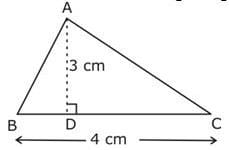Solution:

Area of triangle= ½ *base*height
=½*4*3=6cm2

QUESTION: 4

A door frame of dimensions 4m * 5m is fixed on the wall of dimension 11m * 11m. Find the total labour charges for painting the wall if the labour charges for painting 1m2 of the wall is Rs 2.50.

Solution:
QUESTION: 5

What is the circumference of a circle of diameter 10 cm?

Solution:
QUESTION: 6

Find the breadth of a rectangular plot of land, if its area is 440 m2 and the length is 22 m.

Solution:
QUESTION: 7

The ______ is the distance around a given two-dimensional object.

Solution:
QUESTION: 8

If we cut a square along one of its diagonals, two triangles are obtained. Area of each triangle obtained = __________.

Solution:
QUESTION: 9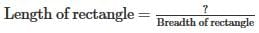Solution:
QUESTION: 10

A rectangular garden is 65 cm long and 50 cm wide. Two cross paths each 2 m wide are to be constructed parallel to the sides. If these paths pass through the centre of the garden, find the cost of constructing the paths at the rate Rs. 69 per m2.

Solution:

The rectangular field is in m and not in cms .
One Path have measurements 65cm and 2cm and its area is 65*2=130m2.
Area of the other path is 50*2=100m2. Total area of the path=130+100=230m2.
Now since the middle square has been counted two times so we will subtract it So, total area to be constructed =230-2*2 =230-4= 226m2.
So Total Cost=226*69=Rs. 15594

QUESTION: 11

The figure given below, shows two circles with the same centre. The radius of the larger circle is 10 cm and the radius of the smaller circle is 4 cm. Find the area of the larger circle.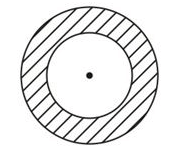Solution:

Area of larger circle = πr2=3.14*100=314cm2

QUESTION: 12

The figure given below, shows two circles with the same centre. The radius of the larger circle is 10 cm and the radius of the smaller circle is 4 cm. Find the area of the smaller circle.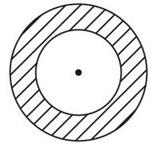Solution:
QUESTION: 13

The figure given below, shows two circles with the same centre. The radius of the larger circle is 10 cm and the radius of the smaller circle is 4 cm. Find the shaded area between the two circles.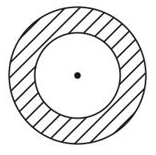Solution:

Area of the larger circle = πR= 3.14*100=314cm2
Area of smaller circle=r2=3.14*16=50.24cm2

QUESTION: 14

A wire is in the shape of a square of side 10 cm. If the wire is bent into a rectangle of length 12 cm, find its breadth. Which encloses more area – the square or the rectangle?

Solution:

Since wire is the boundary which will remain same, we will calculate perimeter of both the shapes
Perimeter of square=4*s=4*10=40cm
Perimeter of rectangle=2(l+b)=2(12+b)
Perimeter of square=Perimeter of rectangle
40cm=24+2b
2b=16
b=8cm
Area of square=10*10=100cm2
Area of rectangle=12*8=96cm2
Therefore, the square has more area.

QUESTION: 15

If the area of the triangle is 36 cm2 and the height is 3 cm, the base of the triangle will be

Solution:

Area of triangle= ½ *base*height
36=½*base*3
36*2=base*3
base=36*⅔=24cm

QUESTION: 16

The base in the area of triangle is

Solution:

Area of triangle = 1/2base x height
2*area=base x height
base=2*area/height

QUESTION: 17

The distance around a circular region is known as its

Solution:
QUESTION: 18

The perimeter of square of side 2.5 m is

Solution:
QUESTION: 19

The perimeter of rectangle of length 1.5 cm and breadth 2 cm is

Solution:
QUESTION: 20

The area of parallelogram whose base 6 cm and altitude 7 cm is

Solution:Use Code STAYHOME200 and get INR 200 additional OFF Use Coupon Code